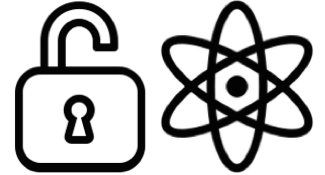# Vibration Modes: Normal modes

## Normal Modes - vibration modes in molecules: molecular topology - classified under Group Theory, distinguishing features: orthogonal to each other, symmetry, and frequencies often predicting wavelength range of active in sample (IR and/or Raman active).

Degrees of Freedom (3N where N is the #nuclei per molecule)

3 coordinates translation motion

3 coordinates rotation motion

3n - m (where m is 6 or 5, pending on if it's non-linear or linear, respectively)

Moleculevmodes
CO24n=3; 3n - 5 = 3(3) - 5
CO1

n=2; 3n - 5 = 3(2) - 5

v = stretching ∆ bond length

δ = bending  ∆ in bond angle

ρr  =  rocking ∆ in angle between group of atoms

ρw = wagging ∆ in angle between plane of group of atoms

ρ = twisting ∆ in angle between planes of two groups of atoms

π = out of plane

s = symmetric

as = symmetric

d = degenerate

https://chem.libretexts.org/Core/Physical_and_Theoretical_Chemistry/Spectroscopy/Vibrational_Spectroscopy/Vibrational_Modes/Normal_Modes

https://chem.libretexts.org/Reference/Reference_Tables/Group_Theory_Tables/Character_Tables

https://en.wikipedia.org/wiki/Molecular_symmetry

A rotation about principal axis = symmetrical

B rotation about principal axis = asymetrical

E & T doubly and triply degenerate

inversion center: g = no change in sign, u = change in sign w/ respect to inversion.

## Character Tables:

CO2 - cyclic Cnv Group ( n-fold proper rotation axis Cn )

E, C2, σ (xz) & σ (yz)

C2vEC2σ (xz)σ (yz)
v11111a1
v21111a2
v31-1-11b2

A1g, b1g, eu = stretching vibrations

b2g, a2u, b2u, eu = bending vibrations

# Quantum rotational angular momentum

## J total angular momentum quantum number

s + l

https://en.wikipedia.org/wiki/Total_angular_momentum_quantum_number

J and branches: P ∆J = -1 Branch, Q - ∆J = 0 Branch, R - ∆J = 1 Branch

https://chem.libretexts.org/Core/Physical_and_Theoretical_Chemistry/Spectroscopy/Rotational_Spectroscopy/Rovibrational_Spectroscopy

Open NanoCarbon and all content is licensed under CC-BY: Creative Commons Attribution 4.0 International License, except where otherwise listed.CC Icons by The Noun Project. Wiki sponsored by Atlassian.